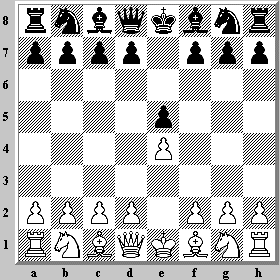## 19 February 2013

### A Pawn Equals 200 Rating Points

Continuing the discussion of Kaufman's Material Imbalances, there are two methods of estimating the value of an imbalance in chess. The first method that all chess players learn is the 1/3/5/9 scale, where the unit is the value of a Pawn. Kaufman frequently resorts to another method, estimating an imbalance in terms of a rating difference. For example, here's a passage from 'The Evaluation of Material Imbalances':-

In case you are wondering what the study showed the rating equivalent of a Pawn to be, I must point out that it is a tricky question. The problem is that when one side is up in material, sometimes it's because he's just outplayed his opponent, but other times it's because the opponent has sacrificed the material for some compensation.

If we make the fair but arbitrary assumption that on average the player who is behind in material has 50% compensation for it, then the rating value of a Pawn (without compensation) works out to about 200 points. In other words, if you outrate your opponent by 200 points but blunder away a Pawn for nothing in the middlegame, the chances should be equal.

In other words, a Pawn is equivalent to a 200 point rating difference. In my page on Chess Ratings, I copy a well known table that equates a 200 point difference to a 0.76, i.e. 76%, chance of winning. To keep it simple, we can round this to 75% for easier mental calculation. The Kaufman paragraph that I just quoted is followed by a discussion of the value of a tempo.

Statistics show that in (international) master play White is worth about 40 rating points; since White's advantage is a half tempo, that means a tempo is worth about 80 points in the opening position. Gambit theory suggests that at the start a Pawn is worth between two and three tempi, so if we use 2 1/2 times 80 we get the same 200 figure.

That squares nicely with a calculation from Kaufman's later article, the one I discussed in One Imbalance Leads to Another. Here a tempo is defined in terms of a Pawn.

• 0.4 - Value of a tempo
• 0.2 - Value of first move

As a practical example, consider the following;Black(!) to move

This diagram is not the result of 1.e4 e5, but rather 1.e3 e5 2.e4. White started with a first move advantage of 0.2 (of a Pawn), then squandered a tempo to lose 0.4, giving him a net result of -0.2, i.e. 0.2 for Black. A lost tempo on White's second move means the first move advantage passes to Black -- exactly what we expect to happen.

***

Later: Another practical example is to combine the observation

• 0.2 - Value of first move [as a fraction of a Pawn]

with

• A Pawn Equals 200 Rating Points

This implies that the value of the first move is 40 rating points (1/5 of a Pawn). Going back to the table from 'Chess Ratings' (rating expectancies vs. differences), a difference of 40 rating points gives the higher rated player a little less than a 0.56 chance of winning the game. This means a 56% expected score for White, and a 44% expected score for Black, which is close to the result derived from databases of historical master-level games.